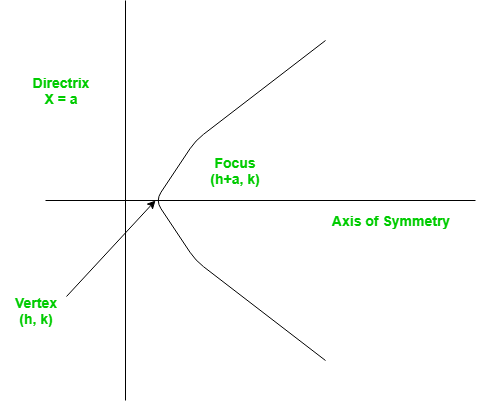# C/C++ Program for Finding the vertex, focus and directrix of a parabola

• Last Updated : 05 Dec, 2018

A set of points on a plain surface that forms a curve such that any point on that curve is equidistant from the focus is a parabola.
Vertex of a parabola is the coordinate from which it takes the sharpest turn whereas a is the straight line used to generate the curve.We provide nothing but the best curated videos and practice problems for our students. Check out the C Foundation Course and master the C language from basic to advanced level. Wait no more, start learning today!

The standard form of a parabola equation is. Given the values of a, b and c; our task is to find the coordinates of vertex, focus and the equation of the directrix.

Example –

```Input : 5 3 2
Output : Vertex:(-0.3, 1.55)
Focus: (-0.3, 1.6)
Directrix: y=-198
Consult the formula below for explanation.
```

## Recommended: Please try your approach on {IDE} first, before moving on to the solution.

 `#include ``using` `namespace` `std;`` ` `// Function to calculate Vertex, Focus and Directrix``void` `parabola(``float` `a, ``float` `b, ``float` `c)``{``    ``cout << ``"Vertex: ("` `<< (-b / (2 * a)) << ``", "``         ``<< (((4 * a * c) - (b * b)) / (4 * a))``         ``<< ``")"` `<< endl;``    ``cout << ``"Focus: ("` `<< (-b / (2 * a)) << ``", "``         ``<< (((4 * a * c) - (b * b) + 1) / (4 * a))``         ``<< ``")"` `<< endl;``    ``cout << ``"Directrix: y="``         ``<< c - ((b * b) + 1) * 4 * a << endl;``}`` ` `// Driver Function``int` `main()``{``    ``float` `a = 5, b = 3, c = 2;``    ``parabola(a, b, c);``    ``return` `0;``}`
Output:
```Vertex: (-0.3, 1.55)
Focus: (-0.3, 1.6)
Directrix: y=-198
```

Please refer complete article on Finding the vertex, focus and directrix of a parabola for more details!

My Personal Notes arrow_drop_up